Miscellaneous

Chapter 13 Class 11 Limits and Derivatives
Serial order wise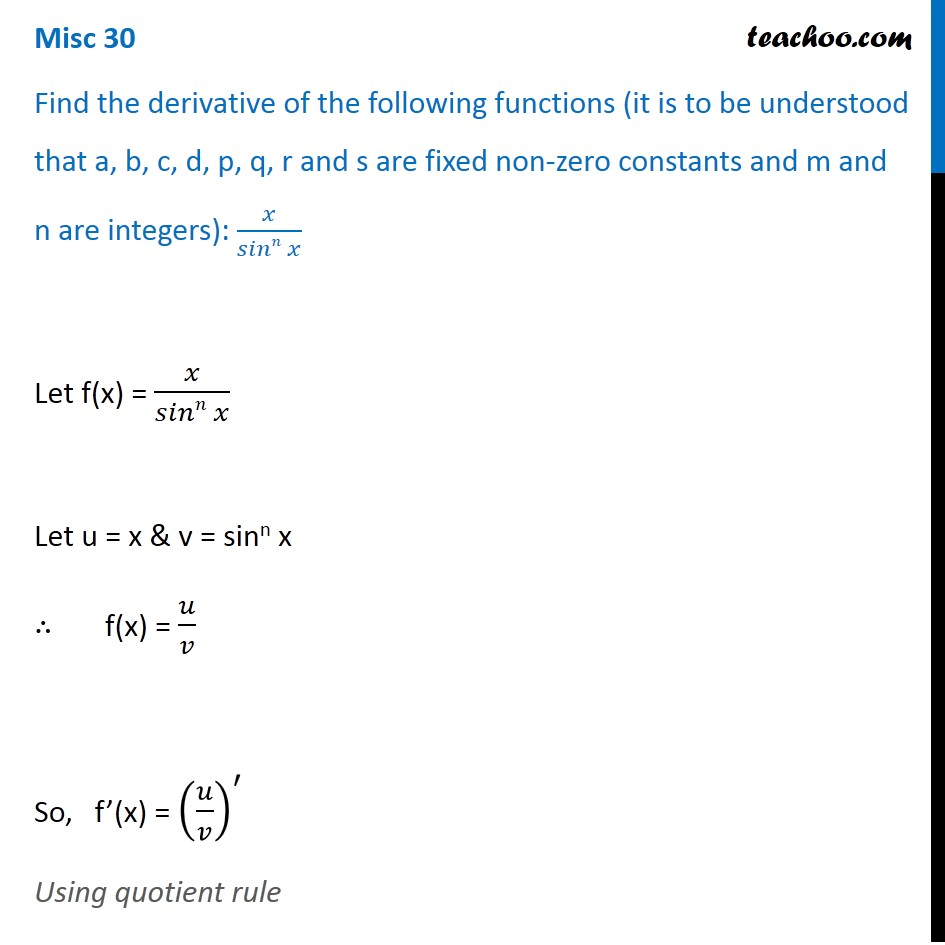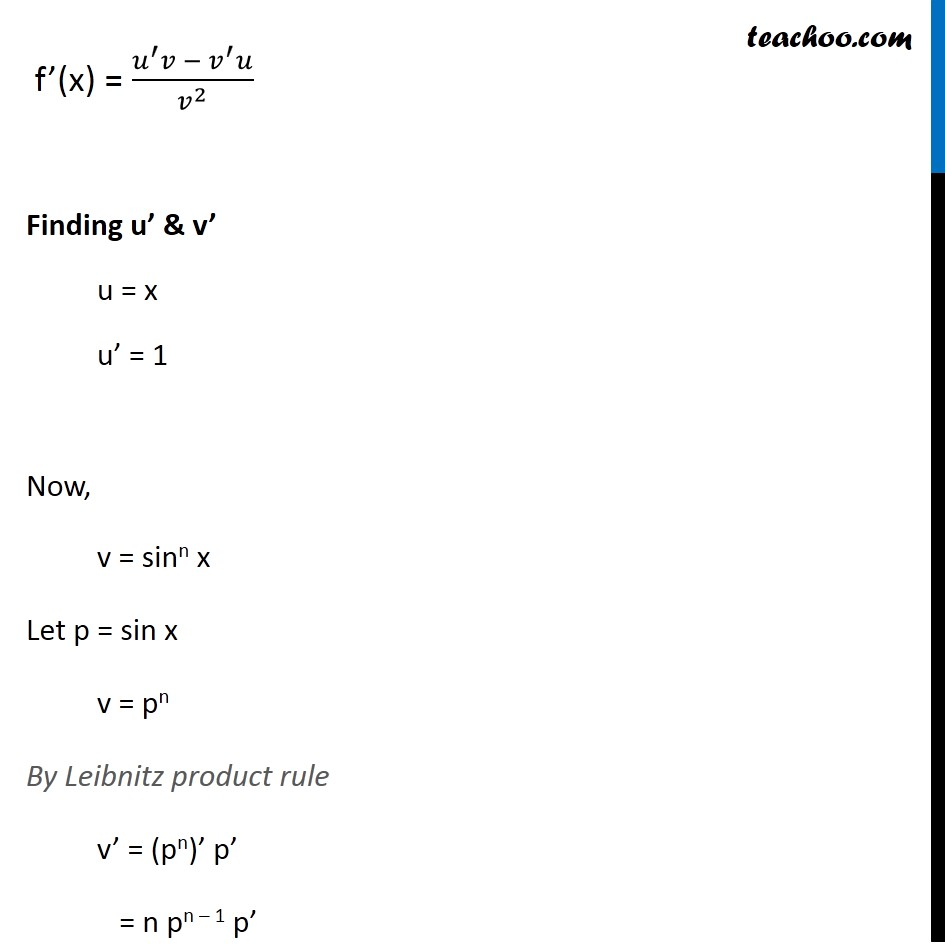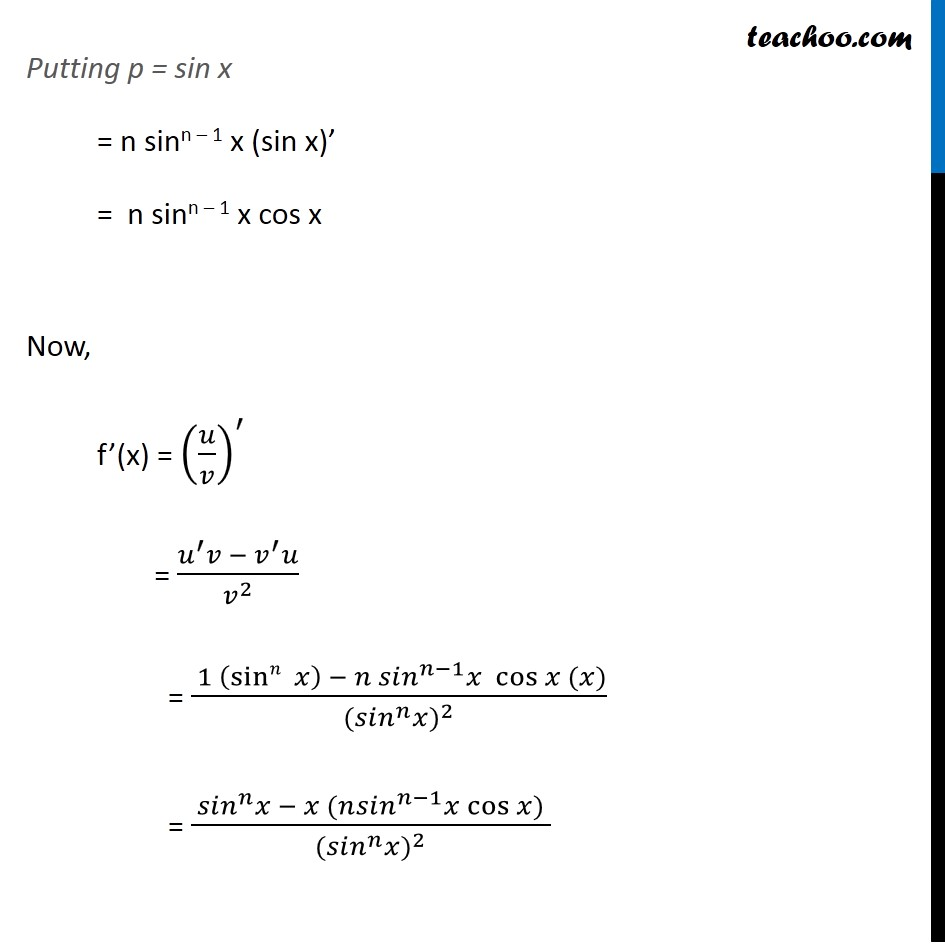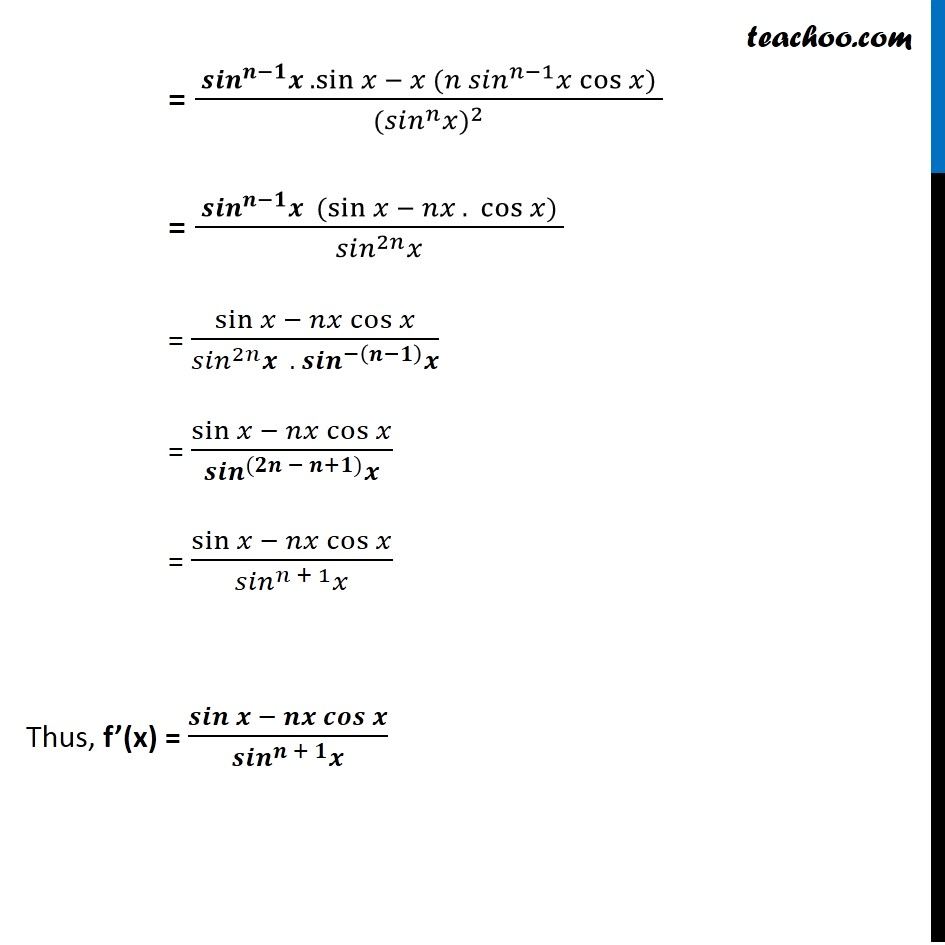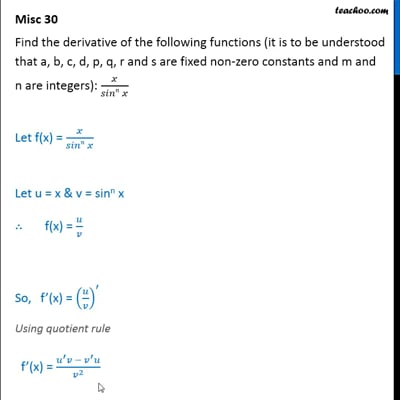This video is only available for Teachoo black users

Introducing your new favourite teacher - Teachoo Black, at only ₹83 per month

### Transcript

Misc 30 Find the derivative of the following functions (it is to be understood that a, b, c, d, p, q, r and s are fixed non-zero constants and m and n are integers): 𝑥/(𝑠𝑖𝑛𝑛 𝑥) Let f(x) = 𝑥/(𝑠𝑖𝑛𝑛 𝑥) Let u = x & v = sinn x ∴ f(x) = 𝑢/𝑣 So, f’(x) = (𝑢/𝑣)^′ Using quotient rule f’(x) = (𝑢^′ 𝑣 −〖 𝑣〗^′ 𝑢)/𝑣^2 Finding u’ & v’ u = x u’ = 1 Now, v = sinn x Let p = sin x v = pn By Leibnitz product rule v’ = (pn)’ p’ = n pn – 1 p’ Putting p = sin x = n sinn – 1 x (sin x)’ = n sinn – 1 x cos x Now, f’(x) = (𝑢/𝑣)^′ = (𝑢^′ 𝑣 −〖 𝑣〗^′ 𝑢)/𝑣^2 = ( 1 (sin𝑛⁡〖 𝑥〗 ) − 〖𝑛 𝑠𝑖𝑛〗^(𝑛−1) 𝑥 cos⁡〖𝑥 (𝑥)〗)/〖〖(𝑠𝑖𝑛〗^𝑛 𝑥)〗^2 = ( 〖𝑠𝑖𝑛〗^𝑛 𝑥 − 𝑥 (𝑛〖𝑠𝑖𝑛〗^(𝑛−1) 𝑥 cos⁡〖𝑥) 〗)/〖〖(𝑠𝑖𝑛〗^𝑛 𝑥)〗^2 = ( 〖𝒔𝒊𝒏〗^(𝒏−𝟏) 𝒙 . sin⁡〖𝑥 − 𝑥 (𝑛 〗 〖𝑠𝑖𝑛〗^(𝑛−1) 𝑥 cos⁡〖𝑥) 〗)/〖〖(𝑠𝑖𝑛〗^𝑛 𝑥)〗^2 = ( 〖𝒔𝒊𝒏〗^(𝒏−𝟏) 𝒙 〖(sin〗⁡〖𝑥 − 𝑛𝑥 . 〗 cos⁡〖𝑥) 〗)/(〖𝑠𝑖𝑛〗^2𝑛 𝑥) = sin⁡〖𝑥 − 𝑛𝑥 cos⁡𝑥 〗/(〖𝑠𝑖𝑛〗^2𝑛 𝒙 . 〖𝒔𝒊𝒏〗^(−(𝒏−𝟏) ) 𝒙) = sin⁡〖𝑥 − 𝑛𝑥 cos⁡𝑥 〗/(〖𝒔𝒊𝒏〗^((𝟐𝒏 − 𝒏+𝟏)) 𝒙) = sin⁡〖𝑥 − 𝑛𝑥 cos⁡𝑥 〗/(〖𝑠𝑖𝑛〗^(𝑛 + 1) 𝑥) Thus, f’(x) = 𝒔𝒊𝒏⁡〖𝒙 − 𝒏𝒙 𝒄𝒐𝒔⁡𝒙 〗/(〖𝒔𝒊𝒏〗^(𝒏 + 𝟏) 𝒙)